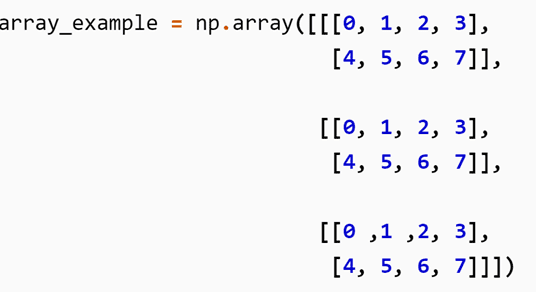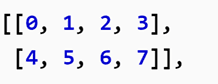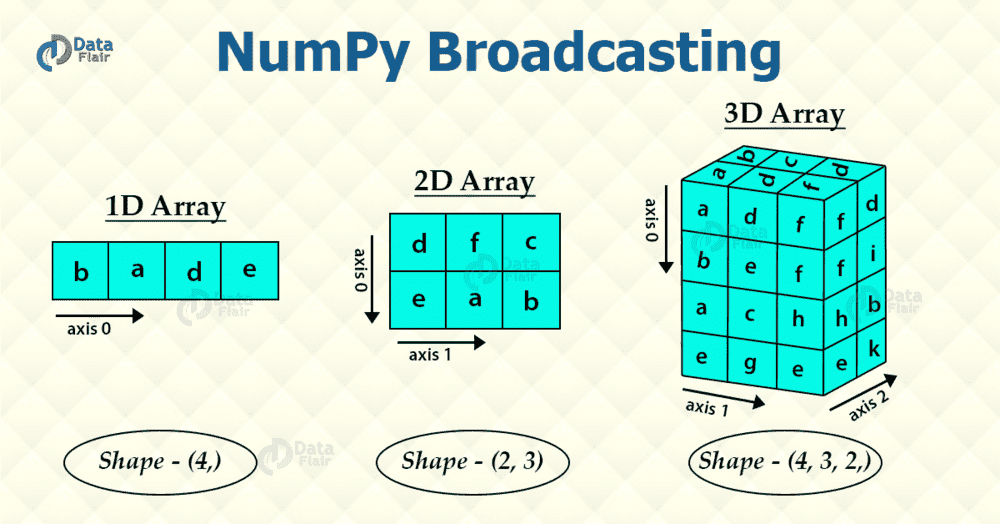# 3D Numpy Array indices....

fog37
TL;DR Summary
3D numpy array indices and array shape
Hello,
I am clear on 1D and 2D Numpy arrays, how to create them and address them).
• 1D array: single list
• 2D array: list containing multiple lists as elements
• 3D array: list containing lists which contain lists as elements
Array elements can be address using indices as a[], a[][], a[][][] respectively.

I have a question about 3D arrays like the one below:I would say that it is a 2x4x3 array but that is not correct when I use the command
Python:
array_example.shape
. The result is (3,2,4). My understanding is that shape should give (row, column, layer). My interpretation is that it is a 3D array composed of 3 identical 2x4 2D arraysThe command shape seem have the first integer in the tuple (, , ) represents the number of layers of the 3D array instead..

Last edited:

Gold Member
2022 Award
In numpy, arrays are "nested" structures. In 2D arrays, you have rows, and under each row, you have columns. So, columns are nested under rows.

Similarly, for 3D arrays, the outermost axes is the "depth" (layer), under which you have rows, and then columns.

Therefore, when you execute np.reshape(array_example), numpy will show you the sizes of the axes from outside-inwards. In your example, there are 3 layers; inside each layer you have 2 rows, and under each row, there are 4 columns.

A bit perplexing. But that's how it works.

Also see this question on Stack Overflow:
https://stackoverflow.com/questions/22981845/3-dimensional-array-in-numpy

Edit: Fixed grammar.

Last edited:
•fog37
fog37
In numpy, arrays are "nested" structures. In 2D arrays, you have rows, and under each row, you have columns. So, columns are nested under rows.

Similarly, for 3D arrays, the outermost axes is the "depth" (layer), under which you have rows, and then columns.

Therefore, when you execute np.reshape(array_example), numpy will show you the sizes of the axes from outside-inwards. In your example, there are 3 layers; inside each layer you have 2 rows, and under each row, there are 4 columns.

A bit perplexing. But that's how it works.

Also see this question on Stack Overflow:
https://stackoverflow.com/questions/22981845/3-dimensional-array-in-numpy

Edit: Fixed grammar.
Thank you. Now I see. It makes sense now.

I read about row-major and column-major in relation to how array data elements are stored linearly in memory. Apparently numpy uses row-major (column indices change the fastest) when the array elements are arranged in line, row by row from top to bottom.

What is the connection between row-major and the fact that the order is (depth, row, column)?
You mention that "...In 2D arrays, you have rows, and under each row, you have columns. So, columns are nested under rows..."

In what sense are columns nested under rows?

fog37
T
The array shape is (4,3,2) which mean 4 arrays (each array is 2x3). So, in 3D arrays, axis 0 is reserved to the depth, axis 1 to rows, and axis 2 to columns: (dim along axis 0, dim along axis 1, dim along axis 2).

the first 2D array is the top onethe first 2D array in the stack is the top one (see below). That would be the first nested list of lists when we define the array. The confusion arises because the axis 0 here counts the layers while in 2D arrays it referred to the rows of 2D arrays.Gold Member
2022 Award
What is the connection between row-major and the fact that the order is (depth, row, column)?
There isn't any connection, as far as I can say. In Numpy, you can specify which style you want to store the array — the C-style (row major) or Fortran(F)-style (column major). In either style, the order of indexing the array will be the same.
You mention that "...In 2D arrays, you have rows, and under each row, you have columns. So, columns are nested under rows..."

In what sense are columns nested under rows?
If you think in terms of memory, everything is flat. There is no nesting of any kind. I said that columns can be thought to be "nested" under rows because that will allow you to remember which comes first.

Simple logic says that new dimensions should be added to the right. I don't understand why the creators of numpy decided to add new dimensions to the left instead. Just results in confusion.

•fog37